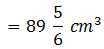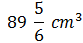Newbie

# A hemispherical bowl has a radius of 3.5 cm. What would be the volume of water it would contain?

• 0

An Important Question of class 10 Based on Mensuration Chapter of M.L Aggarwal for ICSE BOARD.
The radius of a hemispherical bowl is given.
What would be the volume of water it would contain?
This is the Question Number 14, Exercise 17.3 of M.L Aggarwal.

Share

1.Given radius of the hemispherical bowl, r = 3.5 cm = 7/2 cm

Volume of the hemisphere = (2/3)r3

= (2/3)×(22/7)×(7/2)3

= 11×49/6

= 539/6Hence the volume of the hemispherical bowl is.

• 0